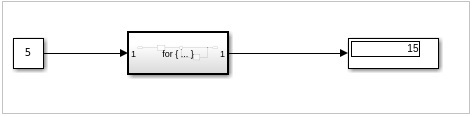# MATLAB Simulink - For Loop

#### Artificial Neural Network and Machine Learning using MATLAB

54 Lectures 4 hours

#### Machine Learning for Data Science using MATLAB

Featured

62 Lectures 9 hours

In this chapter, let us understand the working of for-iterator block. First, create a blank model as shown below −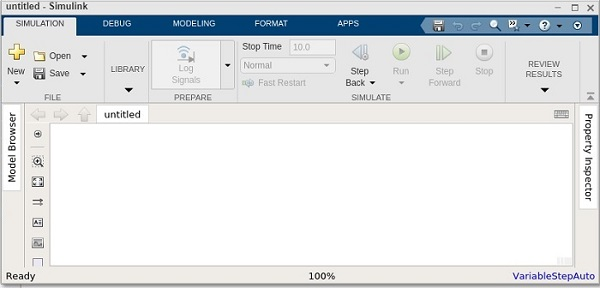In this model, we are going to make use of for iterator that will give us the sum of 1..N.

You can use the value of n as per your choice. This value will take the constant block and update it with value 5 as shown below −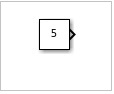Let us add the for-iterator block as shown below −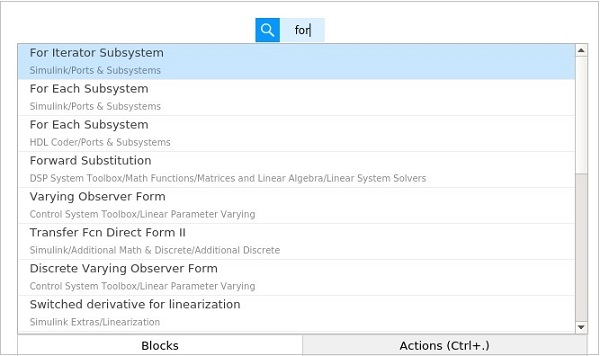Select the for Iterator subsystem block and add to your model. Next, we need display block as shown below −Connect the blocks as shown below −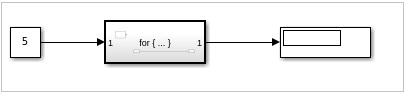The for iterator block is a subsystem. Select the block and click enter. It will take you to new model area, where the for block has to be defined.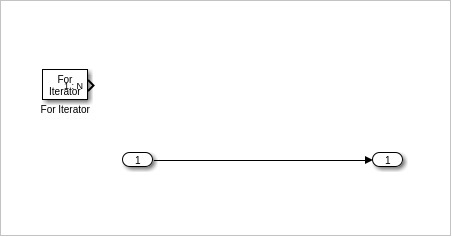Right click on the for iterator and select the block parameters, as shown below −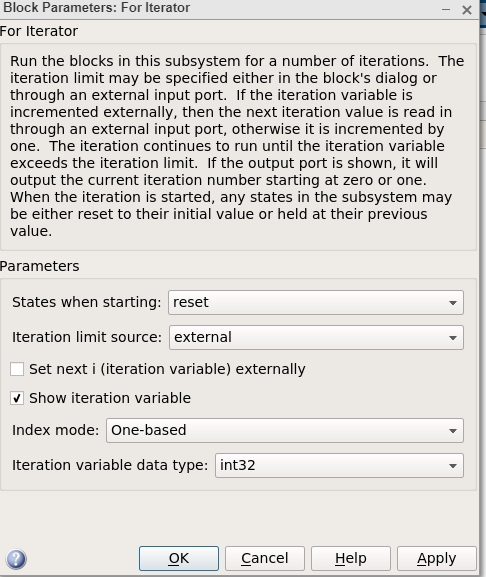Change the States when starting as reset and Iteration limit source as external. Click on Ok to update the changes.

Now, you will get an input block to your for loop, as given below −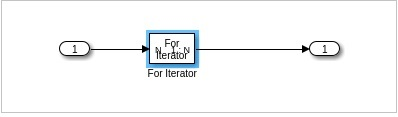We need a sum block and a delay block as shown below −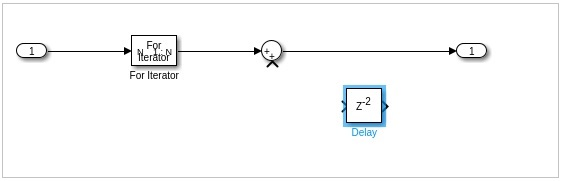The delay block has to be flipped so that it can be added to the output. We need to give the output back to the sum block so that it can be added with the current iteration.

Right click on delay block and change the delay length from 2 to 1 as shown below. Click on OK to update the changes.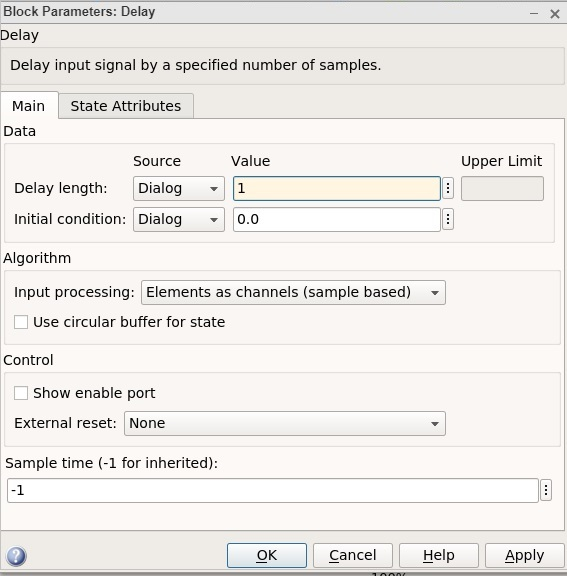The final for-loop subsystem block will look as follows −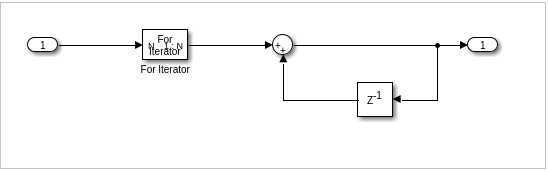Now before you run the simulation, change the stop time to 1. We do this because we want the simulation to run only once.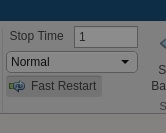Click on Run now to see the result in display block as shown below

The input value is 5, so the for-loop will go from 1 to 5. Hence, the values 1+2+3+4+5 = 15 is shown in the display.